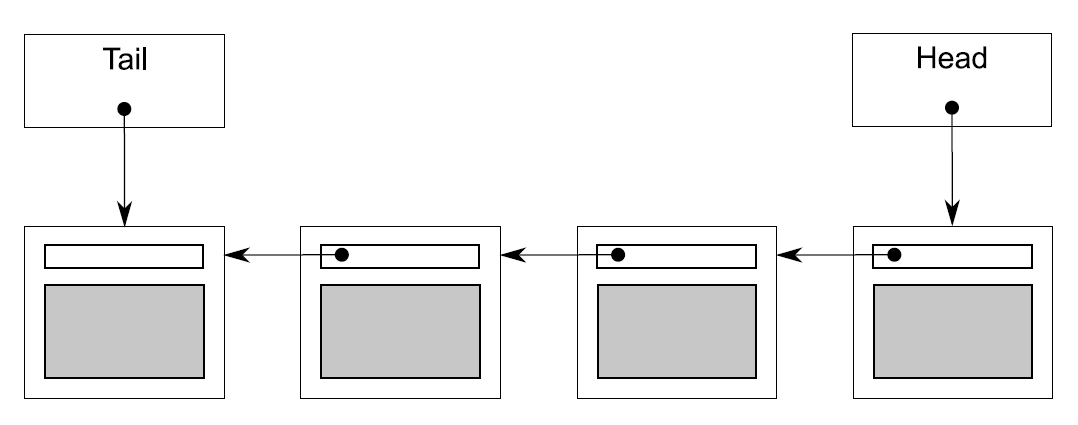6.2 基于锁的并发数据结构

6.2.1 线程安全栈——使用锁

#include <exception>

struct empty_stack: std::exception
{
const char* what() const throw();
};

template<typename T>
{
private:
std::stack<T> data;
mutable std::mutex m;
public:
{
std::lock_guard<std::mutex> lock(other.m);
data=other.data;
}

void push(T new_value)
{
std::lock_guard<std::mutex> lock(m);
data.push(std::move(new_value));  // 1
}
std::shared_ptr<T> pop()
{
std::lock_guard<std::mutex> lock(m);
if(data.empty()) throw empty_stack();  // 2
std::shared_ptr<T> const res(
std::make_shared<T>(std::move(data.top())));  // 3
data.pop();  // 4
return res;
}
void pop(T& value)
{
std::lock_guard<std::mutex> lock(m);
if(data.empty()) throw empty_stack();
value=std::move(data.top());  // 5
data.pop();  // 6
}
bool empty() const
{
std::lock_guard<std::mutex> lock(m);
return data.empty();
}
};

6.2.2 线程安全队列——使用锁和条件变量

template<typename T>
{
private:
mutable std::mutex mut;
std::queue<T> data_queue;
std::condition_variable data_cond;

public:
{}

void push(T new_value)
{
std::lock_guard<std::mutex> lk(mut);
data_queue.push(std::move(data));
data_cond.notify_one();  // 1
}

void wait_and_pop(T& value)  // 2
{
std::unique_lock<std::mutex> lk(mut);
data_cond.wait(lk,[this]{return !data_queue.empty();});
value=std::move(data_queue.front());
data_queue.pop();
}

std::shared_ptr<T> wait_and_pop()  // 3
{
std::unique_lock<std::mutex> lk(mut);
data_cond.wait(lk,[this]{return !data_queue.empty();});  // 4
std::shared_ptr<T> res(
std::make_shared<T>(std::move(data_queue.front())));
data_queue.pop();
return res;
}

bool try_pop(T& value)
{
std::lock_guard<std::mutex> lk(mut);
if(data_queue.empty())
return false;
value=std::move(data_queue.front());
data_queue.pop();
return true;
}

std::shared_ptr<T> try_pop()
{
std::lock_guard<std::mutex> lk(mut);
if(data_queue.empty())
return std::shared_ptr<T>();  // 5
std::shared_ptr<T> res(
std::make_shared<T>(std::move(data_queue.front())));
data_queue.pop();
return res;
}

bool empty() const
{
std::lock_guard<std::mutex> lk(mut);
return data_queue.empty();
}
};

wiat_and_pop()函数是等待队列向栈进行输入的一个解决方案；比起持续调用empty()，等待线程调用wait_and_pop()函数和数据结构处理等待中的条件变量的方式要好很多。对于data_cond.wait()的调用，直到队列中有一个元素的时候，才会返回，所以你就不用担心会出现一个空队列的情况了，还有，数据会一直被互斥锁保护。因为不变量这里并未发生变化，所以函数不会添加新的条件竞争或是死锁的可能。

template<typename T>
{
private:
mutable std::mutex mut;
std::queue<std::shared_ptr<T> > data_queue;
std::condition_variable data_cond;
public:
{}

void wait_and_pop(T& value)
{
std::unique_lock<std::mutex> lk(mut);
data_cond.wait(lk,[this]{return !data_queue.empty();});
value=std::move(*data_queue.front());  // 1
data_queue.pop();
}

bool try_pop(T& value)
{
std::lock_guard<std::mutex> lk(mut);
if(data_queue.empty())
return false;
value=std::move(*data_queue.front());  // 2
data_queue.pop();
return true;
}

std::shared_ptr<T> wait_and_pop()
{
std::unique_lock<std::mutex> lk(mut);
data_cond.wait(lk,[this]{return !data_queue.empty();});
std::shared_ptr<T> res=data_queue.front();  // 3
data_queue.pop();
return res;
}

std::shared_ptr<T> try_pop()
{
std::lock_guard<std::mutex> lk(mut);
if(data_queue.empty())
return std::shared_ptr<T>();
std::shared_ptr<T> res=data_queue.front();  // 4
data_queue.pop();
return res;
}

void push(T new_value)
{
std::shared_ptr<T> data(
std::make_shared<T>(std::move(new_value)));  // 5
std::lock_guard<std::mutex> lk(mut);
data_queue.push(data);
data_cond.notify_one();
}

bool empty() const
{
std::lock_guard<std::mutex> lk(mut);
return data_queue.empty();
}
};

std::shared_ptr<>持有数据的好处：新的实例分配结束时，不会被锁在push()⑤当中(而在清单6.2中，只能在pop()持有锁时完成)。因为内存分配操作的需要在性能上付出很高的代价(性能较低)，所以使用std::shared_ptr<>的方式对队列的性能有很大的提升，其减少了互斥量持有的时间，允许其他线程在分配内存的同时，对队列进行其他的操作。

6.2.3 线程安全队列——使用细粒度锁和条件变量template<typename T>
class queue
{
private:
struct node
{
T data;
std::unique_ptr<node> next;

node(T data_):
data(std::move(data_))
{}
};

node* tail;  // 2

public:
queue()
{}
queue(const queue& other)=delete;
queue& operator=(const queue& other)=delete;
std::shared_ptr<T> try_pop()
{
{
return std::shared_ptr<T>();
}
std::shared_ptr<T> const res(
return res;
}

void push(T new_value)
{
std::unique_ptr<node> p(new node(std::move(new_value)));
node* const new_tail=p.get();
if(tail)
{
tail->next=std::move(p);  // 4
}
else
{
}
tail=new_tail;  // 6
}
};

template<typename T>
class queue
{
private:
struct node
{
std::shared_ptr<T> data;  // 1
std::unique_ptr<node> next;
};

node* tail;

public:
queue():
{}
queue(const queue& other)=delete;
queue& operator=(const queue& other)=delete;

std::shared_ptr<T> try_pop()
{
{
return std::shared_ptr<T>();
}
return res;  // 6
}

void push(T new_value)
{
std::shared_ptr<T> new_data(
std::make_shared<T>(std::move(new_value)));  // 7
std::unique_ptr<node> p(new node);  //8
tail->data=new_data;  // 9
node* const new_tail=p.get();
tail->next=std::move(p);
tail=new_tail;
}
};

template<typename T>
{
private:
struct node
{
std::shared_ptr<T> data;
std::unique_ptr<node> next;
};
std::mutex tail_mutex;
node* tail;

node* get_tail()
{
std::lock_guard<std::mutex> tail_lock(tail_mutex);
return tail;
}

{
{
return nullptr;
}
}
public:
{}

std::shared_ptr<T> try_pop()
{
}

void push(T new_value)
{
std::shared_ptr<T> new_data(
std::make_shared<T>(std::move(new_value)));
std::unique_ptr<node> p(new node);
node* const new_tail=p.get();
std::lock_guard<std::mutex> tail_lock(tail_mutex);
tail->data=new_data;
tail->next=std::move(p);
tail=new_tail;
}
};

• tail->next == nullptr

• tail->data == nullptr

• 在列表中的每一个节点x，x!=tail且x->data指向一个T类型的实例，并且x->next指向列表中下一个节点。x->next == tail意味着x就是列表中最后一个节点

{
node* const old_tail=get_tail();  // ① 在head_mutex范围外获取旧尾节点的值

{
return nullptr;
}
}

OK，所以清单6.6提供了一个使用细粒度锁的线程安全队列，不过只有try_pop()可以并发访问(且只有一个重载存在)。那么在清单6.2中方便的wait_and_pop()呢？你能通过细粒度锁实现一个相同功能的接口吗？

template<typename T>
{
private:
struct node
{
std::shared_ptr<T> data;
std::unique_ptr<node> next;
};

std::mutex tail_mutex;
node* tail;
std::condition_variable data_cond;
public:
{}

std::shared_ptr<T> try_pop();
bool try_pop(T& value);
std::shared_ptr<T> wait_and_pop();
void wait_and_pop(T& value);
void push(T new_value);
void empty();
};

template<typename T>
{
std::shared_ptr<T> new_data(
std::make_shared<T>(std::move(new_value)));
std::unique_ptr<node> p(new node);
{
std::lock_guard<std::mutex> tail_lock(tail_mutex);
tail->data=new_data;
node* const new_tail=p.get();
tail->next=std::move(p);
tail=new_tail;
}
data_cond.notify_one();
}

template<typename T>
{
private:
node* get_tail()
{
std::lock_guard<std::mutex> tail_lock(tail_mutex);
return tail;
}

{
}

std::unique_lock<std::mutex> wait_for_data()  // 2
{
}

{
}

{
}
public:
std::shared_ptr<T> wait_and_pop()
{
}

void wait_and_pop(T& value)
{
}
};

template<typename T>
{
private:
{
{
return std::unique_ptr<node>();
}
}

{
{
return std::unique_ptr<node>();
}
}
public:
std::shared_ptr<T> try_pop()
{
}

bool try_pop(T& value)
{
}

void empty()
{